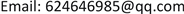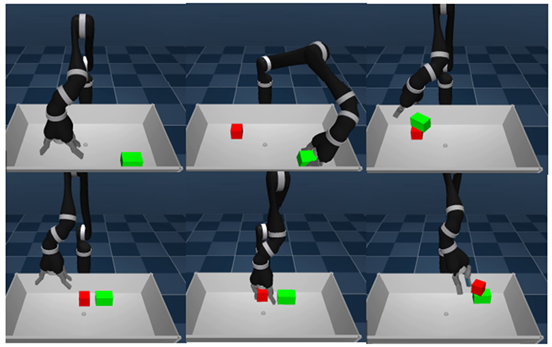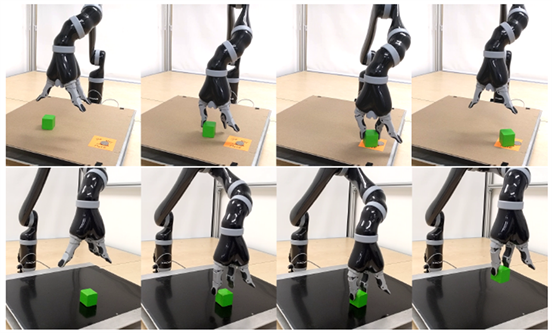﻿ 基于深度强化学习的机械臂视觉抓取控制优化方法 Visual Grasp Control Optimization Method and System for Manipulator Based on Deep Reinforcement Learning

Artificial Intelligence and Robotics Research
Vol. 07  No. 04 ( 2018 ), Article ID: 27782 , 7 pages
10.12677/AIRR.2018.74024

Visual Grasp Control Optimization Method and System for Manipulator Based on Deep Reinforcement Learning

Bang Lin, Wei Zhou, Jiangbo Fan, Rui Li

Zhaotong Power Supply Bureau, Yunnan Power Grid Co., Ltd., Zhaotong YunnanReceived: Nov. 6th, 2018; accepted: Nov. 22nd, 2018; published: Nov. 29th, 2018ABSTRACT

In order to improve the compatibility between visual image features and optimal control, this paper proposes an optimization method for visual grasp control of manipulator arm based on deep reinforcement learning, which can extract features from visual images generated by interaction with the environment independently and directly apply the extracted features. By continuously grasping the experiment and optimizing the manipulator arm grabbing strategy, an excellent grasping strategy is finally obtained. And we can learn the grasping control strategy of the manipulator according to different grasping tasks and grasping environment.

Keywords:Deep Learning, Manipulator Arm, Machine Vision, Grasping Strategy1. 引言

2. 深度学习神经网络模块设计

2.1. 执行器–评价器结构

2.2. 卷积神经网络

${F}_{t}=Convs\left({o}_{t};{\theta }_{t}\right)$

${H}_{t}=\left\{\begin{array}{l}0\text{\hspace{0.17em}}\text{\hspace{0.17em}}\text{\hspace{0.17em}}\text{\hspace{0.17em}}\text{\hspace{0.17em}}\text{\hspace{0.17em}}\text{\hspace{0.17em}}\text{\hspace{0.17em}}\text{\hspace{0.17em}}\text{\hspace{0.17em}}\text{\hspace{0.17em}}\text{\hspace{0.17em}}\text{\hspace{0.17em}}\text{\hspace{0.17em}}\text{\hspace{0.17em}}\text{\hspace{0.17em}}\text{\hspace{0.17em}}\text{\hspace{0.17em}}\text{\hspace{0.17em}}\text{\hspace{0.17em}}\text{\hspace{0.17em}}\text{\hspace{0.17em}}\text{\hspace{0.17em}}\text{\hspace{0.17em}}\text{\hspace{0.17em}}\text{\hspace{0.17em}}\text{\hspace{0.17em}}\text{\hspace{0.17em}}\text{\hspace{0.17em}}\text{\hspace{0.17em}}\text{\hspace{0.17em}}t=0\\ LSTM\left({F}_{t}^{f},{H}_{t-1}|{\theta }_{t}\right)\text{\hspace{0.17em}}\text{\hspace{0.17em}}\text{\hspace{0.17em}}\text{\hspace{0.17em}}0

${P}_{t}=Softmax\left({O}^{R}|{\theta }_{t}\right)$

${V}_{t}=Linear\left({O}^{R}|{\theta }_{t}\right)$

3. 控制优化方法及实例

3.1. 学习流程Figure 1. Learning processFigure 2. Neural network structure

3.2. 控制策略

3.2.1. 贝尔曼方程

${G}_{t}={R}_{t+1}+\gamma {R}_{t+2}+\cdots =\underset{k=0}{\overset{\infty }{\sum }}{\gamma }^{k}{R}_{t+k+1}$

$v\left(s\right)=E\left[{G}_{t}|{S}_{t}=s\right]$

$\begin{array}{c}v\left(s\right)=E\left[{G}_{t}|{S}_{t}=s\right]\\ =E\left[{R}_{t+1}+\gamma {R}_{t+2}+{\gamma }^{2}{R}_{t+3}+\cdots |{S}_{t}=s\right]\\ =E\left[{R}_{t+1}+\gamma \left({R}_{t+2}+\gamma {R}_{t+3}+\cdots \right)|{S}_{t}=s\right]\\ =E\left[{R}_{t+1}+\gamma {G}_{t+1}|{S}_{t}=s\right]\\ =E\left[{R}_{t+1}+\gamma \left({S}_{t+1}\right)|{S}_{t}=s\right]\end{array}$

3.2.2. 神经网络参数选取

3.3. 抓取控制实例Figure 3. Example of grab control of the robot arm in the simulation environmentFigure 4. Example of grab control of a robot arm in a real environment

4. 结束语

Visual Grasp Control Optimization Method and System for Manipulator Based on Deep Reinforcement Learning[J]. 人工智能与机器人研究, 2018, 07(04): 200-206. https://doi.org/10.12677/AIRR.2018.74024

1. 1. 曾毅, 刘成林, 谭铁牛. 类脑智能研究的回顾与展望[J]. 计算机学报, 2016, 39(1): 212-222.

2. 2. 朱虎明, 李佩, 焦李成, 等. 深度神经网络并行化研究综述[J]. 计算机学报, 2018, 41(8): 1861-1881.

3. 3. Kang, L., Ye, P., Li, Y. and Doermann, D. (2014) Convolutional Neural Networks for no-Reference Image Quality Assessment. Proceedings of the IEEE International Conference on Computer Vision and Pattern Recognition, Columbus, 23-28 June 2014, 1733-1740. https://doi.org/10.1109/CVPR.2014.224

4. 4. Ren, S.Q., He, K.M., Girshick, R., et a1. (2015) Faster R-CNN: Towards Re-al-Time Object Detection with Region Proposal Networks. Proceedings of the Neural Information and Processing System, Montreal, 7-12 December 2015, 91-99.

5. 5. 马玉芳. 基于智能视觉的微型高精度图像采集系统设计[J]. 现代电子技术, 2018, 41(19): 67-70.

6. 6. 孔峻. 深度卷积神经网络在计算机视觉中的应用[J]. 电子技术与软件工程, 2018(21): 130-131.

7. 7. 王曌, 胡立生. 基于深度Q学习的工业机械臂路径规划方法[J]. 化工自动化及仪表, 2018(2): 141-145.

8. 8. 李文博. 基于视觉伺服的机械臂控制系统研究[D]: [硕士学位论文]. 南京: 南京理工大学, 2016.

9. 9. 李宇飞, 高朝辉, 申麟. 基于视觉的机械臂空间目标抓取策略研究[J]. 中国科学: 技术科学, 2015, 45(1): 31-35.

10. 10. 王军南, 丛爽. 基于视觉定位的二自由度机械臂控制系统[J]. 机械与电子, 2008(2): 49-52.

11. 11. 杨马英, 葛轶众. 基于BP神经网络的机械臂视觉伺服控制[J]. 计算机应用, 2017(a02): 279-282.

12. 12. 白成超, 晏卓, 宋俊霖. 结合深度学习的机械臂视觉抓取控制[J]. 载人航天, 2018, 21(3): 299-307.

13. 13. Redmon, J., Divvala, S., Girshick, R., et al. (2016) You Only Look Once: Unified, Real-Time Object Detection. IEEE Conference on Computer Vision and Pattern Recognition, Las Vegas, 26-30 June 2016, 779-788. https://doi.org/10.1109/CVPR.2016.91

14. 14. Redmon, J. and Farhadi, A. (2016) YOLO9000: Better, Faster, Stronger. 2017 IEEE Conference on Computer Vision and Pattern Recognition, Honolulu, 21-26 July 2017, 6517-6525.

15. 15. 薛天. 深度强化学习原理及其在机器人运动控制中的运用[J]. 通讯世界, 2018(8): 240-241.

16. 16. 任秉银, 魏坤, 吴卓琦. 机械臂视觉伺服路径规划研究进展[J]. 哈尔滨工业大学学报, 2018, 50(1): 1-10.

17. 17. 陈学松, 杨宜民. 基于执行器-评价器学习的自适应PID控制[J]. 控制理论与应用, 2011, 28(8): 1187-1192.